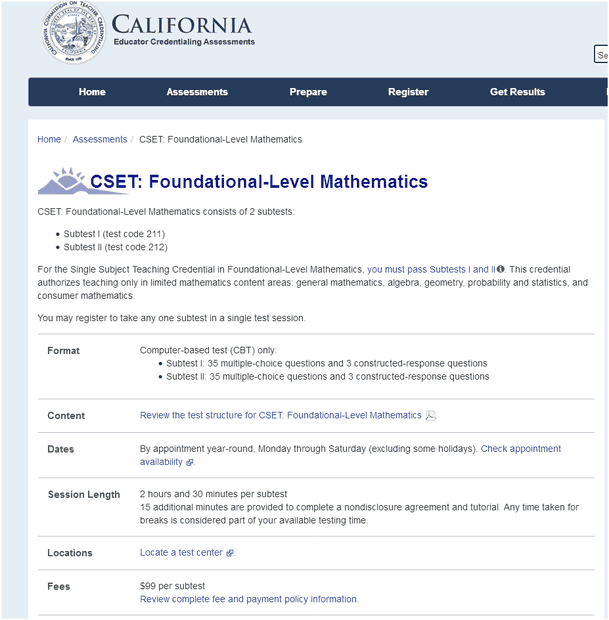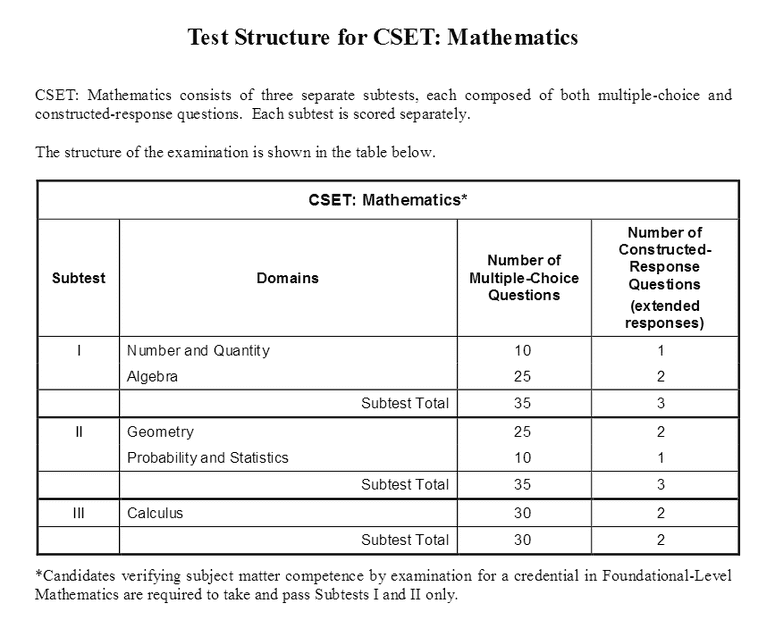# Foundational Mathematics

Homework Helper
Gold Member
I had seen some online information many years ago or some literature that I did not track saying that Introductory Algebra and Geometry such as the two courses at the high school level are "Foundational Mathematics Courses". I wonder if what I read was misstated, or if these Foundational courses are restricted to only those two? Why is maybe, Intermediate Algebra not also considered "Foundational Mathematics" course? Too, why should Trigonometry be left out?

•berkeman

Gold Member
2022 Award
Seems to me it's just a matter of where you draw the line. It would be perfectly reasonable to me to say that arithmetic is foundational math since without it you can't do algebra.

OR algebra and geometry are foundational because without them you can't do trig.

OR algebra and trig and geometry are foundational because ...

•Astronuc
Homework Helper
Gold Member
Seems to me it's just a matter of where you draw the line. It would be perfectly reasonable to me to say that arithmetic is foundational math since without it you can't do algebra.

OR algebra and geometry are foundational because without them you can't do trig.

OR algebra and trig and geometry are foundational because ...
Whatever I was reading, I believed I was reading from something official and formal.

Gold Member
2022 Award
Whatever I was reading, I believed I was reading from something official and formal.
"official" according to whom?

Homework Helper
Gold Member
Whatever I was reading, I believed I was reading from something official and formal.
Not sure where you are from but in the US each state has its own high school curriculum standards, this is also true for each constituent country (England, Scotland, Wales and Northern Ireland) of the UK (I don't know about any other countries). So there is no generally relevant answer to your question.

Homework Helper
Gold Member
"official" according to whom?
"Official" according to any formal governing state and local organizations or districts.

Homework Helper
Gold Member
Not sure where you are from but in the US each state has its own high school curriculum standards, this is also true for each constituent country (England, Scotland, Wales and Northern Ireland) of the UK (I don't know about any other countries). So there is no generally relevant answer to your question.
Whatever I was reading was whatever I was reading. At this time, I have no tracking for what or where exactly I read it. If some state, XYZ, in some country has some official statement that some named courses are Foundational Courses of a subject then that is what I meant by "official".

Homework Helper
Gold Member
In that case how is it possible to answer your original questions?

Gold Member
2022 Award
"Official" according to any formal governing state and local organizations or districts.
And you think all such organizations agree on such "definitions". Good luck with that.

Mentor

https://www.ctcexams.nesinc.com/TestView.aspx?f=HTML_FRAG/CA_CSET212_TestPage.htmlhttps://www.ctcexams.nesinc.com/content/docs/CSET_Prep/CS_mathematics_geninfo.pdfHomework Helper
Gold Member
Post #10 -- That Test Structure for CSET: Mathematics table is very helpful. It makes Foundational Mathematics look like Algebra, Geometry, Calculus; and that the credentialing test expects certain kinds of candidates to be proficient with the Algebra and the Geometry parts.

It seems, @berkeman found what I had read so many years ago. Not know if it was recently revised but reflects the information I though I found.

•berkeman
Mentor
found what I had read so many years ago. Not know if it was recently revised but reflects the information I though I found.
I'm guessing that they did revise it at least to add Calculus in. I think you would have remembered that part if it was in what you originally read. It seems like a nice addition, IMO.Homework Helper
Gold Member
I'm guessing that they did revise it at least to add Calculus in. I think you would have remembered that part if it was in what you originally read. It seems like a nice addition, IMO.Clear logical thoughts.

Time then that some people may need to heavily review all of their Calculus 1 and Calculus 2 concepts and skills. This can make Subject Matter Qualifications for some educators more difficult these days.

•berkeman
Without a firm foundation in basic arithmetic, algebras (I, II) and geometry a student will struggle in precalculus and above.

The following programs reflect what I believe is meant by foundational math.

From the University of New Mexico

http://news.unm.edu/news/foundational-math-taking-fear-anxiety-out-of-learning-mathematics

The course is called Foundational Math (Univ. 103) and is a division of University College’s Academic Foundations Program. Primarily computer-based, the class is designed for students who need brush up on some general math and algebra concepts before going into higher level courses required for most majors.

https://www.ccp.edu/college-catalog/course-offerings/foundational-mathematics-courses

Courses in:
arithmetic
elementary algebra
Introductory Mathematics
intermediate algebra
Retail Mathematics & Merchandising

Sonoma State University

https://education.sonoma.edu/programs/FLM

Foundational Level Mathematics Teaching Credential program.

The FLMTC authorizes teaching general mathematics, all levels of algebra, probability and statistics, geometry, and consumer mathematics, but not calculus or mathematics analysis.

https://files.eric.ed.gov/fulltext/EJ1068208.pdf

A College-Level Foundational Mathematics Course: Evaluation,
Challenges, and Future Directions

All three courses cover basic number facts and operations—addition, subtraction,
multiplication, and division—with rational numbers, including positive and negative integers
and fractions; linear algebraic equations; the order of operations—brackets, exponents, division,
multiplication, addition, and subtraction; ratio, proportion, and percent; basic graphical
techniques; and, basic manipulation of equations containing variables. The level of difficulty of
the GAS course is comparable to that of the Ontario grade eight curriculum while the CV and
CV+ course are at a grade six level.

Staff Emeritus
I had seen some online information many years ago or some literature that I did not track saying that Introductory Algebra and Geometry such as the two courses at the high school level are "Foundational Mathematics Courses". I wonder if what I read was misstated, or if these Foundational courses are restricted to only those two? Why is maybe, Intermediate Algebra not also considered "Foundational Mathematics" course? Too, why should Trigonometry be left out?
I would agree with Algebra and Geometry being foundational, but so is Arithmetic.

Trigonometry is a subset of Geometry. One person puts it: "Geometry is a superset of trigonometry, whereas trigonometry is a subset of geometry."

Someone else states:"geometry is the study of different shapes, sizes, and positions of different shapes based on the number of sides, angles, and so on. Whereas trigonometry is the subset of geometry that deals with the properties of one of the shapes in geometry called “Triangle”."
https://www.vedantu.com/maths/difference-between-trigonometry-and-geometry

A Wikipedia article on generalized geometry mentions, "trigonometry can be the study of triples of points in any kind of geometry or space. A triangle is the polygon with the smallest number of vertices, . . ."
https://en.wikipedia.org/wiki/Generalized_trigonometry

In my junior high (middle) school and high school programs, I took courses: Algebra I (Grade 9), Geometry and Trigonometry (Grade 10), Algebra II (Grade 11) and Analytical Geometry and Calculus (Grade 12). In Grade 10, I was in honors math class. We did the year long Geometry class in one semester, and followed with a year long Trigonometry class in the second semester. The Algebra II, Analytical Geometry and Analysis/Calculus (the course was called Calculus, but began with analysis) were done on a Trimester basis at a different high school in the same city public school system. The math and science program at the second high school was much better than that of the first high school. The course in Algebra II involved trigonometry and introductory analysis in preparation for the Calculus course.

More digression - In Grades 11, I did honors Chemistry (I and II), and in Grade 12, I did honors Physics. We actually started doing differential equations in the Chemistry I class based on rate equations and solubility problems. The teachers of the honors math and chemistry programs worked together with the same group of students. The year I took Physics, we had a new teacher who was still green. The math teachers were active in the American Mathematical Society, and the chemistry teacher (MS in Chemistry) was active in the American Chemical Society, and had left a research position to teach high school.

I would visit the AMS website and look for articles on the foundations of mathematics - https://www.ams.org/home/page
or something equivalent in other nations, e.g.,
https://smf.emath.fr/, https://france.math.cnrs.fr/
https://www.mathematik.de/en
https://www.mathunion.org/imu-members/united-kingdom
https://euro-math-soc.eu/
https://www.mathsoc.jp/en/
and many more

### Thirty Years of Foundational Studies Lectures on the Development of Mathematical Logic and the Study of the Foundations of Mathematics in 1930–1964​

https://www.sciencedirect.com/science/article/abs/pii/S0049237X09704586

Someone's perspective - https://en.wikipedia.org/wiki/Foundations_of_mathematics
https://en.wikipedia.org/wiki/Grundlagen_der_Mathematik

https://www.amazon.com/dp/1942270755/?tag=pfamazon01-20

Last edited:
•berkeman
Homework Helper
Gold Member
Astronuc, such finely detailed well-thought examining the subject, but the original question relates to how some governing boards (like in Education) arrange the so very many topics and subtopics, and of the competency testing of those "Foundational Courses".

About your earlier part of your post talking about Arithmetic - some people say that Algebra (like Algebra 1 and 2) is just Arithmetic using variables.

Staff Emeritus
About your earlier part of your post talking about Arithmetic - some people say that Algebra (like Algebra 1 and 2) is just Arithmetic using variables.
Yes and no. Arithmetic is the branch of mathematics dealing with the properties and manipulation of numbers. I think of basic arithmetic being something like counting numbers, as in 0, 1, 2, 3, . . . . , then operations such as addition/subtraction and multiplication/division, then exponentiation and roots.

https://en.wikipedia.org/wiki/Arithmetic

Algebra is a "broad area of mathematics, together with number theory, geometry and analysis. In its most general form, algebra is the study of mathematical symbols and the rules for manipulating these symbols; it is a unifying thread of almost all of mathematics."
Ref: https://en.wikipedia.org/wiki/Algebra

"Elementary algebra differs from arithmetic in the use of abstractions, such as using letters to stand for numbers that are either unknown or allowed to take on many values." Wikipedia: Algebra

I'm curious about the taxonomy of mathematics, because teachers in primary education generally do not go into the broader picture of the field of math, ostensibly because it would be way over the heads of most students, and possibly most teachers in the lower grades.

Homework Helper
Gold Member
I'm curious about the taxonomy of mathematics, because teachers in primary education generally do not go into the broader picture of the field of math, ostensibly because it would be way over the heads of most students, and possibly most teachers in the lower grades.
That is a very important general idea. I do not have the patience to give much discussion on it at the moment.
All I could say about it is, Some students learning Mathematics in K-8, not good; Some of those same students if study Algebra 1 and Algebra 2 in high school, much much better.

Those who say algebra is just generalized arithmetic shouldn't be making decisions about a math curriculum. They probably got lost in the transition from the prescriptive approach of arithmetic to the more analytical approach of algebra. School arithmetic only deals with rational numbers. Irrational and complex numbers arise in the more general dealing with relationships that are developed in algebra, not in arithmetic. Algebra is the student's first encounter with real mathematics and the need to learn a more cognitive approach to learning. There is a transition from ordinary arithmetic to algebra when equality is not just the result of a computation but related to the more general concept of equivalence.

.

•Mark44 and phinds
Homework Helper